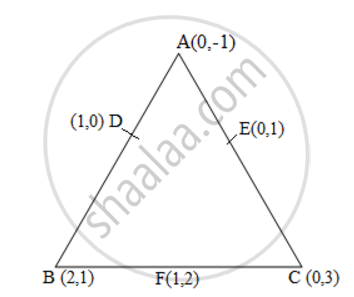# Find the area of the triangle formed by joining the mid-points of the sides of the triangle whose vertices are (0, -1), (2, 1) and (0, 3). Find the ratio of this area to the area of the given triangle - Mathematics

Find the area of the triangle formed by joining the mid-points of the sides of the triangle whose vertices are (0, -1), (2, 1) and (0, 3). Find the ratio of this area to the area of the given triangle

#### SolutionLet the vertices of the triangle be A (0, -1), B (2, 1), C (0, 3).

Let D, E, F be the mid-points of the sides of this triangle. Coordinates of D, E, and F are given by

D = (0+2/2 , -1+1/2) = (1,0)

E = (0+0/2 , -3-1/2) = (0,1)

F = (2+0/2 , 1+3/2) = (1,2)

Area of a triangle = 1/2 {x1 (y2 - y3) + x2 (y3 - y1) + x3 (y1 - y2)}

Area of ΔDEF = 1/2 {1(2-1) + 1(1-0) + 0(0-2)}
= 1/2 (1+1) = 1 square units

Area of ΔABC = 1/2 [0(1-3) + 2{3-(-1)} + 0(-1-1)]
= 1/2 {8} = 4 square units

Therefore, the required ratio is 1:4.
Concept: Area of a Triangle
Is there an error in this question or solution?
Chapter 7: Coordinate Geometry - Exercise 7.3 [Page 170]

#### APPEARS IN

NCERT Class 10 Maths
Chapter 7 Coordinate Geometry
Exercise 7.3 | Q 3 | Page 170
Share